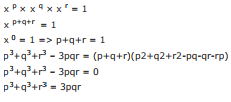Courses

# Number System - MCQ 3

## 20 Questions MCQ Test Quantitative Ability for SSC CHSL | Number System - MCQ 3

Description
This mock test of Number System - MCQ 3 for Quant helps you for every Quant entrance exam. This contains 20 Multiple Choice Questions for Quant Number System - MCQ 3 (mcq) to study with solutions a complete question bank. The solved questions answers in this Number System - MCQ 3 quiz give you a good mix of easy questions and tough questions. Quant students definitely take this Number System - MCQ 3 exercise for a better result in the exam. You can find other Number System - MCQ 3 extra questions, long questions & short questions for Quant on EduRev as well by searching above.
QUESTION: 1

### In the examination a candidate must get 2/5 marks to pass, out of total marks.Shiyam appeared in the exam ang got 198 marks and still failed by 36 marks.The maximum mark is

Solution:

2x = 198+36 = 234
Max = 234*5/2 = 585

QUESTION: 2

### If 4 is added to the numerator of a fraction it becomes 1/3 and if 3 is added to the denominator it becomes 1/6 then find the numerator and denominator is

Solution:

5/27 = 9/27 = 1/3
5/30 = 1/6

QUESTION: 3

### One-third of a 2 digit number exceeds its one-fifth by 8.What is the 2 digit number ?

Solution:

1/3(10x+y) -1/5(10x+y) = 8
(10x+y)[5/15 – 3/15] = 8
10x+y = 8*15/2 = 60

QUESTION: 4

12 +22 +……………………………………+242

Solution:

Sum of square number = n(n+1)(2n+1)/6
N = 24
Sum = 24*25*49/6 = 4900

QUESTION: 5

Micael gets 3 marks for each correct questions and loses 2 marks for each wrong answers.He attempts 30 sum and obtain 30 marks.Find the no of Questions he answered correctly ?

Solution:

18*3 – 12*2 = 54-24 = 30

QUESTION: 6

What half of a number is added to 234, it becomes five time of itself. What is the number ?

Solution:

x/2 + 234 = 5x
5x – x/2 = 234
9x/2 = 234
X = 234*2/9= 52

QUESTION: 7

Twenty times a positive integer is less than its square by 96. What is the Integer ?

Solution:

X2 – 20x = 96
(x-24)(x+4) = 0
X = 24

QUESTION: 8

There are some Parrots and Some Lions in a forest.If the total number of animals head in forest are 840 and total no of animal legs are 1760.What is the number of Parots in the forest ?

Solution:

2x+4(840-x) = 1760
2x+3360-4x = 1760
-2x = 1600
X = 1600/2 = 800

QUESTION: 9

When all the students in a school are made to stand in row of 68, 40 such rows are formed.If the students are made to stand in the row of 20, how many such rows can be formed ?

Solution:

No of row = 68*40/20 = 136

QUESTION: 10

A banana costs Rs.1.25 and Orange costs Rs2.75. What will be the total cost of 10 bananas and 2 dozens Orange ?

Solution:

1.25*10 +2.75*24 = 12.5 +66 = 78.50

QUESTION: 11

What will be the Unit digit of 27!

Solution:

1*2*3*………………………………26*27
Factorial after 6 should end with 0

QUESTION: 12

xp × xq × xr = 1 then p3 + q3 + r3 is equal to

Solution:QUESTION: 13

If the divisor is five times the quotient and six times the remainder, if the remainder is 5 then the dividend is

Solution:

Q=6, R = 5
Dividend = divisor*Q + R
Dividend = 30*6 + 5 = 180+5 = 185

QUESTION: 14

If the sum and the product of 2 numbers are 25 and 144 respectively then the difference of the number should be

Solution:

A+B = 25
AB = 24
(a-b)2 = (a+b)2 – 4ab
(a-b)2 = 25 – 4(144)
(a-b)2 = 625 – 576
(a-b)2 = 49
a-b = 7

QUESTION: 15

The sum of the two digit number is 11. The number obtained by reversing the digit is 27 less than the original number.Then the original number is

Solution:

4+7 = 11
74-47 = 27

QUESTION: 16

The product of 2 consecutive even number is 1224, then one of the number is

Solution:

34*36 = 1224

QUESTION: 17

212 +222 +232 +…………………302 = ?

Solution:

12 +22 +32 +…………………n2 = 1/6[n(n+1)(2n+1)]
= 30*31*61/6 – 20*21*41/6
= 9455 – 2870 = 6585

QUESTION: 18

A number when divided by 361 gives a remainder 47. If the Same number is divided by 19 ,the remainder is

Solution:

47÷19 ⇒ remainder 9
Ex : 769 ÷ 361 ⇒ remainder 47
769 ÷ 19 ⇒ remainder 9

QUESTION: 19

The respective ratio between the two digit of a number is 5:3, if the digit at the one’s place is twice the digit at ten’s place.What is the number ?

Solution:

2x/x = 5/3
6x = 5x

QUESTION: 20

N is the smallest number which when added to the 2000 makes the resulting number divisible by 12, 16, 18 and 21, Then the N is

Solution:

LCM of 12, 16, 18, 21 = 1008
1008*2 = 2016
N = 16